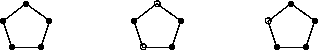Colourings of the n Gon

### Colourings of the n Gon

Let us now consider an application of the preceding results to the paradigmatic G-set YX corresponding to given Y and GX. This yields a nice proof of a famous number theoretic result.
Example: Let Cp denote the following cyclic subgroup of sup:
Cp:= á(1 ...p) ñ <= S p.
It acts on the set X:= p= {1, ...,p } and hence, see the paragigmatic examples, also on the set YX:= m p, which can be considered as the set of all the colourings of the regular p-gon in m colours. For example, in the case when p:=5 and m=2, C 5 acts on the set 2 5, consisting of all the 32 colourings of the regular pentagon with two colours (black and white), some of which are shown in the figure below.Three colourings of the regular 5-gon.

We now assume that p is a prime. The Lemma shows that C p contains, besides the identity element, p-cycles only. The identity element of C p keeps each f Î m p fixed, while each p-cycle fixes the m monochromatic colourings only (notice that (1 ...p) acts as a clockwise rotation of the p-gon after having numbered the vertices of the p-gon from 1 to p, counterclockwise). Hence we obtain from the Cauchy-Frobenius Lemma that

| Cp\\mp | = (1)/(p)(mp+(p-1)m),
provided that p is a prime number. This implies that mp+(p-1)m, and hence also mp-m, is congruent zero modulo p, for each positive integer m. It is clear that this is then also true for any integer z. In the case when z is not divisible by p, we may even divide the difference zp-z by z, obtaining
Fermat's Congruence If p is a prime number and z an integer which is prime to p, then
zp-1 º1 (p).

This shows that group actions can also be useful in elementary number theory, and it seems appropriate to emphasize the following immediate implication of the Cauchy-Frobenius Lemma:

Corollary: For any action of a finite group G on a finite set X we have the congruence
åg ÎG | Xg | º0 ( | G | ).
Later on we shall return to this result and we shall refine it considerably (numbers will be replaced by polynomials, and the congruence will be a congruence for the coefficients of the monomial summands). It is a very helpful tool for number theoretic purposes and shows again the efficiency of finite group actions.
harald.fripertinger@kfunigraz.ac.at,
last changed: August 28, 2001Colourings of the n Gon